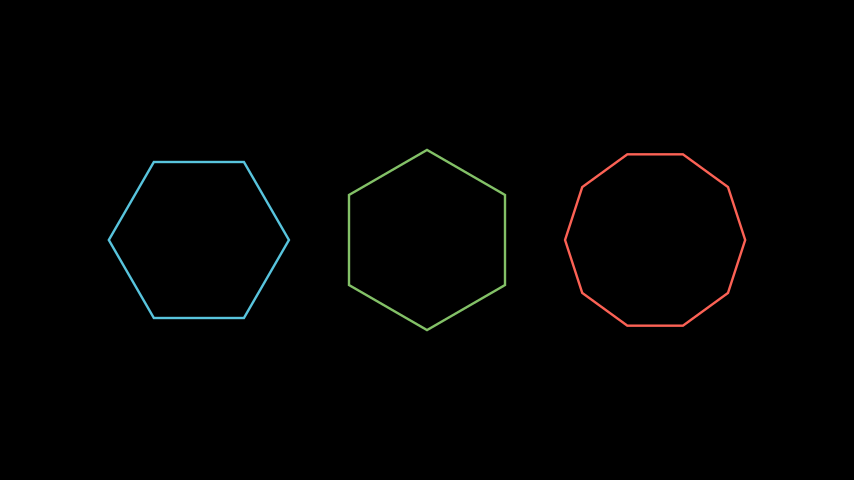# RegularPolygon#

Qualified name: `manim.mobject.geometry.polygram.RegularPolygon`

class RegularPolygon(n=6, **kwargs)[source]#

An n-sided regular `Polygon`.

Parameters

Examples

Example: RegularPolygonExample```from manim import *

class RegularPolygonExample(Scene):
def construct(self):
poly_1 = RegularPolygon(n=6)
poly_2 = RegularPolygon(n=6, start_angle=30*DEGREES, color=GREEN)
poly_3 = RegularPolygon(n=10, color=RED)

poly_group = Group(poly_1, poly_2, poly_3).scale(1.5).arrange(buff=1)
 `animate` Used to animate the application of any method of `self`. `animation_overrides` `color` `depth` The depth of the mobject. `fill_color` If there are multiple colors (for gradient) this returns the first one `height` The height of the mobject. `n_points_per_curve` `sheen_factor` `stroke_color` `width` The width of the mobject.